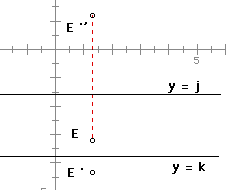# Twice Reflected over Parallel LinesLines y = j and y = k are parallel.
E is an arbitrary point between the two lines.
Reflect point E over line y = k to create point E’.
Reflect point E ‘ over line y = j to create point E”.
Find an equation that relates the distance between E and E”.